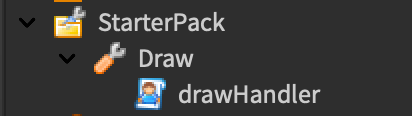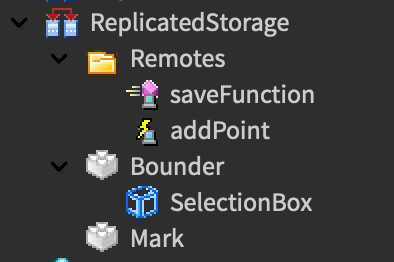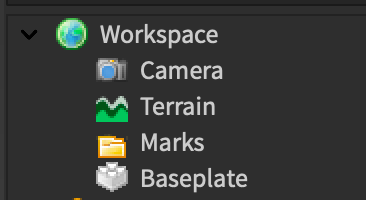# Creating a physical representation of magnitude bounds

Background
I’m creating a drawing game for fun (Won’t ever be public, I know it’s against ToS) to practice applying math to programming and to practice serializing values for DataStores.

Issue
I’ve set the limit on how far a user can draw from to 10 (meaning the magnitude of the position of the player’s root part minus the point on the floor where the player wants to create the point has to be less than or equal to 10) and I’ve decided to have a client-sided box appear when the player equips the tool to show them how far they can draw from. However, as you’ll see in the clip below, the algorithm I’m currently using to find the limits of the magnitude requirement (multiplying the max distance by two on the X and Z coordinates and creating a selection box) is not accounting for diagonal lines. The script prints the magnitude whenever the distance is too far to draw.

Clip

I’m wondering how I can visually represent the limits of the magnitude requirement on the player’s screen.

The Explorer/Code That I’m Using

ExplorerCode
``````local uis = game:GetService("UserInputService")
local players = game:GetService("Players")
local rs = game:GetService("ReplicatedStorage")

local remotes = rs:WaitForChild("Remotes")
local saveFunction = remotes.saveFunction

local myPoints = {}
local inputting = false
local inputHeight = 0.1

local maxDist = 10
local plr = players.LocalPlayer
local mouse = plr:GetMouse()
local tool = script.Parent
local equipped = false

local bounder = rs:WaitForChild("Bounder"):Clone()
bounder.Parent = workspace.CurrentCamera
bounder.Size = Vector3.new(maxDist * 2, bounder.Size.Y, maxDist * 2) -- multiply by two because i don't know why but it seemed to make it more accurate

local function toFloor(vector)
return Vector3.new(vector.X, inputHeight, vector.Z)
end

tool.Equipped:Connect(function()
print("Equipped")
bounder.SelectionBox.Visible = true
equipped = true
end)
tool.Unequipped:Connect(function()
print("Unequipped")
bounder.SelectionBox.Visible = false
equipped = false
end)

uis.InputBegan:Connect(function(inputObject)
if inputObject.UserInputType == Enum.UserInputType.MouseButton1 then
inputting = true
end
end)
uis.InputEnded:Connect(function(inputObject)
if inputObject.UserInputType == Enum.UserInputType.MouseButton1 then
inputting = false
end
end)

game:GetService("RunService").Heartbeat:Connect(function()
local myPos = toFloor(plr.Character.HumanoidRootPart.Position)
bounder.Position = Vector3.new(myPos.X, .025, myPos.Z)
if inputting and equipped then
local frame = toFloor(mouse.Hit.Position) -- find target position of point on set Y axis
local far = (frame - myPos).Magnitude -- calculate magnitude
if not table.find(myPoints, frame) and far <= maxDist then -- make sure no point has been placed there already and that the magnitude is within the limit
table.insert(myPoints, frame)
addPoint:FireServer(frame) -- adds the point on server side (shouldn't affect my issue)
elseif far > maxDist then
print(far) -- this would print the magnitude, as you saw in the video
end
end
end)
``````

Any help is appreciated, thank you!

Thinking about this logically, almost every edge point on a square to the center of the square would have a different magnitude. This is not intuitive for your situation, because we want every edge point of our shape should have the same distance to the center of our shape.

Instead of a square, the shape which could accomplish this task is a circle. Every circle has a radius, ie: the distance from the center of the circle to any edge point on the circle. If the distance of every edge point on the circle to the center point of the circle is the circle’s radius and the circle only has one radius we know for certain then that a circle would then be a valid shape for this problem.

1 Like